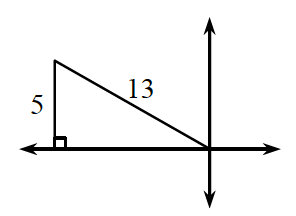### Home > PC3 > Chapter 3 > Lesson 3.2.3 > Problem3-127

3-127.

If $\sin(\theta)=\frac{5}{13}$ and $\frac{\pi}{2}\leθ\le\pi$, sketch a triangle to match this situation and then evaluate $\cos(θ)$ and $\tan(θ)$.

Draw and label a right triangle in the correct quadrant.

Calculate the length of the unknown side.
(Or you may know it because it is a Pythagorean Triple!) When you label it, think about if it should be positive or negative.

From the side lengths, you can determine cos(θ) and tan(θ).